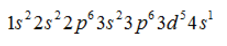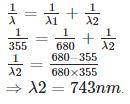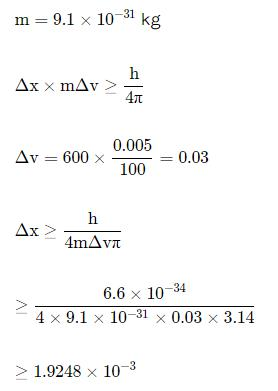Courses

# Test Level 1: Structure Of Atom- 2

## 25 Questions MCQ Test Chemistry for JEE | Test Level 1: Structure Of Atom- 2

Description
This mock test of Test Level 1: Structure Of Atom- 2 for JEE helps you for every JEE entrance exam. This contains 25 Multiple Choice Questions for JEE Test Level 1: Structure Of Atom- 2 (mcq) to study with solutions a complete question bank. The solved questions answers in this Test Level 1: Structure Of Atom- 2 quiz give you a good mix of easy questions and tough questions. JEE students definitely take this Test Level 1: Structure Of Atom- 2 exercise for a better result in the exam. You can find other Test Level 1: Structure Of Atom- 2 extra questions, long questions & short questions for JEE on EduRev as well by searching above.
QUESTION: 1

### Spin quantum number with two spin states of the electron represented by two arrows, ↑ (spin up) and ↓ (spin down) was introduced to account for

Solution:

In anomalous Zeeman effect the splitting of spectral lines occurs due to interaction of the applied magnetic field with the magnetic moment generated by the orbital and 'spin' motions of the electron.

QUESTION: 2

### Electronic configuration of the element having atomic number 24.

Solution:

atomic no 24 is of Cr. Due to half filled orbital stability it doesnot follow Afbau rule so its configuration isQUESTION: 3

### Values of e/m (charge/mass) in the categories alpha particle (α), electron (e) and protons (p) increase in the order:

Solution:

e/m values of particle (α), electron (e) and protons (p) increase in the order a< p < e

QUESTION: 4

A gas absorbs a photon of 355 nm and emits at two wavelengths. If one of the emissions is at 680 nm, the other is at:

Solution:QUESTION: 5

Correct expression for mass number A is

Solution:

mass no. = no. of protons + no. of neutrons.

QUESTION: 6

According to quantum mechanics ψ2(r) the wave function squared gives

Solution:

ψ has no physical significance while ψ2 represnts the probability density of finding an electron.

QUESTION: 7

The electronic configuration 1s22s22p1 belongs to

Solution:

It is of boron as it has atomic number 5.

QUESTION: 8

The atom with the given atomic number Z=17, and the atomic mass A=35.5 is

Solution:

the element with atomic number(Z) 17 & mass number (A) 35 is chlorine = 35/17 cl

QUESTION: 9

The energy associated with the first orbit in the hydrogen atom is -2.18 x 10−18 J/atom. What is the energy associated with the fifth orbit?

Solution:

Energy for any n shell = -2.18 x 10−18 )/n'2 (') stands for raise to the power of therefore, E associated with 5 orbit = -2.18 x 10−18/52 = -2.18 × 10-18/25 = -218 × 10-20/25 = -8.72 x 10-20J/atom

QUESTION: 10

Isobars are the atoms with

Solution:

Isobars are the atoms with same mass number but different atomic number.

QUESTION: 11

Due to the presence of electrons in the inner shells, the electron in the outer shell will not experience the full positive charge of the nucleus (Ze). This is known as

Solution:

This is known as shielding effect. Inner lying electrons shields the positive charge reaching the outer most electrons.

QUESTION: 12

Maximum number of electrons in a subshell with = 3 and = 4 is

Solution:

In this question l=3 means the sub shell is f & n=4 means it is present in 4th orbit.
Finally it is a 4f sub shell.
The no of orbital in f sub shell =2l+1
= 2*3+1
=7
Each orbital can accommodate 2 electrons.
7*2 = 14 electrons.

Maximum number of electrons in a sub shell with l=3& n=4 (4f) is 14electrons.

QUESTION: 13

Arrange the electrons present in the 4d, 3d, 4p and 3p orbitals in order of increasing energies

Solution:

Energy of orbital is determined by n+l value. The orbital having higher n+l value will have higher energy. If n+l value is same then orbital having higher n value will have higher energy.

QUESTION: 14

Isotopes are the atoms with

Solution:

Isotopes have same atomic no. but different mass no.

QUESTION: 15

The Aufbau principle states : In the ground state of the atoms, the orbitals are filled in order of

Solution:

Acc. To aufbau rule electrons are filled in increasing order of energy.

QUESTION: 16

Radio frequency region of the electromagnetic spectrum is used for broadcasting. It is

Solution:

Radio frequency region is present around 106106 Hz.

QUESTION: 17

In an atom, an electron is moving with a speed of 600m/s with an accuracy of 0.005%. Certainity with which the position of the electron can be located is (h = 6.6 ×10−34 Js)

Solution:QUESTION: 18

Give the name and atomic number of the inert gas atom in which the total number of d-electrons is equal to the difference between the numbers of total p and total s electrons.

Solution:

It is Krypton .
Electronic configuration:  1s22s22p63s23p64s23d104p6
​Number of electrons in s orbital are 8
Number of electrons in p orbital are 18
Number of electrons in d orbitals are 10 (18-8=10)

QUESTION: 19

Photoelectric effect established that light

Solution:

Photoelectric effect proves the particle nature of light.

QUESTION: 20

According to Pauli’s exclusion principle

Solution:

Paulis exclusion principle stated that no two electron can have same set of all the four quantum nos.

QUESTION: 21

One of the orbitals is non-directional

Solution:

s orbital is spherically symmetrical hence non directional.

QUESTION: 22

The ionization energy of the lowest state, also called as the ground state, is:

Solution:

for hydrogen atom =  2.18 x 10−18J atom−1 =−E1(H)

QUESTION: 23

An cation A3+ has 18 electrons. Write the atomic number of A.

Solution:

3+ charge means no of electrons will be 3 less than no. of protons. So no. of protons will be 21. So atomic no. is 21.

QUESTION: 24

Emission spectrum of a material results from the material's (atom or molecules)

Solution:

emission spectrum is recorded when a electron de excites from higher energy level to ground state.

QUESTION: 25

Dalton's atomic theory could not explain one of the following

Solution:

It does not account for subatomic particles: Dalton’s atomic theory stated that atoms were indivisible. However, the discovery of subatomic particles (such as protons, electrons, and neutrons) disproved this postulate.